# Class 10 Science: Chapter 12 electricity Exercise Questions|NCERT Solutions

In this page we have Class 10 Science: Chapter 12 electricity Exercise Questions|NCERT Solutions . Hope you like them and do not forget to like , social share and comment at the end of the page.
Question 1.
A piece of wire of resistance R is cut into five equal parts. These parts are then connected in parallel. If the equivalent resistance of this combination is R ′ , then the ratio R/R ′ is –
(a) 1/25
(b) 1/5
(c) 5
(d) 25
Correct option is (d)
Resistance of a piece of wire is proportional to its length. According to question we have a piece of wire of resistance R. The wire is cut into five equal parts. Therefore resistance of each part would be
$R_{new} = \frac {R}{5}$
Since $R = \rho \frac {l}{A}$
All five resistance are connected in parallel. So, for parallel resistance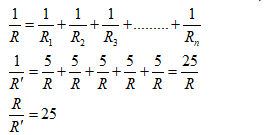Therefore the ratio of R/R’ is 25.

Question 2.
Which of the following terms does not represent electrical power in a circuit?
(a) I2R
(b) IR2
(c) VI
(d) V2/R
the rate at which electric work is done or the rate at which electric energy is consumed is called electric power.
Electric power is given by the expression
$P=VI$ --- (i)
According to Ohm’s Law
$V=IR$--- (ii)
Where,
V = Potential difference
I = Current
R = resistance
Equation (i) can be written as
$P=(IR)I=I^2R$
Again considering equation (ii)
$I=\frac {V}{R}$
$P=VI =V \times \frac {V}{R}= \frac {V^2}{R}$
Power can not be expressed as IR2.

Question 3.
An electric bulb is rated 220 V and 100 W. When it is operated on 110 V, the power consumed will be – (a) 100 W (b) 75 W (c) 50 W (d) 25 W
(d) Power of an appliance determine the rate at which the electrical energy is delivered to it.
Energy consumed by an appliance is given by the expression
$P=VI = \frac {V^2}{R}$
or
$R= \frac {V^2}{P}$
Where,
Power rating, P=100 W
Voltage, V=220 V
Resistance,
$R= \frac {220^2}{100}=484 \Omega$ The resistance of the bulb remains constant if the supply voltage is reduced to 110 V.
If the bulb is operated on 110 V, then the energy consumed by it is given by the expression for power as
$P=\frac {V^2}{R}= \frac {110^2}{484}=25W$ Therefore , the power consumed would be 25 W.

Question 4.
Two conducting wires of the same material and of equal lengths and equal diameters are first connected in series and then parallel in a circuit across the same potential difference. The ratio of heat produced in series and parallel combinations would be –
(a) 1:2 (b) 2:1 (c) 1:4 (d) 4:1
It is given that resistors are equal.
So, for series combination
$R_S=R+R=2R$ Power in series combination is
$P=\frac {V^2}{2R}$
For parallel combination of resistances
$\frac {1}{R_p}= \frac {1}{R} + \frac {1}{R}=\frac {2}{R}$
or
$R_p= \frac {R}{2}$
Power in parallel combination is
$P_p=\frac {V^2}{R/2}= \frac {2V^2}{R}$
So,
$P_s: P_p=\frac {1}{2} : 2 = 1 : 4$

Question 5.
How is a voltmeter connected in the circuit to measure the potential difference between two points?
To measure the potential difference between two points, a voltmeter should be connected in parallel to the points. Also its positive terminal should be connected to the point at higher potential and negative terminal to the point at lower potential.

Question 6.
A copper wire has diameter 0.5 mm and resistivity of 1.6 × 10–8 ? m. What will be the length of this wire to make its resistance 10 ?? How much does the resistance change if the diameter is doubled?
Given that
D=0.5 mm,$r=\frac {.5}{2} \times 10^{-3} m$
$\rho = 1.6 \times 10^{-8} \Omega m$ and $R=10 \Omega$
Using
$R= \frac {\rho l}{A}$
We get
$l= \frac {R \pi r^2}{\rho}$
Since $A=\pi r^2$
$l = \frac {10 \times 3.14}{1.6 \times 10^{-8}} \times (\frac {.5}{2} \times 10^{-3})^2 = 122.6 m=123 m$
If the diameter is doubled radius of copper wire is also doubled.
$\frac {A_1}{A_2} = \frac {\pi r^2}{\pi (2r)^2} = \frac {1}{4}$
So,
$A_2 = 4 A_1$
For the same length and same material wire,
$R \; \alpha \; \frac {1}{A}$
We get
$\frac {R_2}{R_1} = \frac {A_2}{A_1} = \frac {A_1}{4A_1} = \frac {1}{4}$
Therefore, $R_2 = \frac {1}{4} R_1$
Hence, the resistance becomes one fourth of the original one.

Question 7.
The values of current I flowing in a given resistor for the corresponding values of potential difference V across the resistor are given below –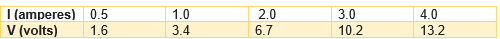Plot a graph between V and I and calculate the resistance of that resistor.
The plot between voltage and current is called VI characteristic. The voltage is plotted on y-axis and current is plotted on x-axis.
The VI graph is plotted as below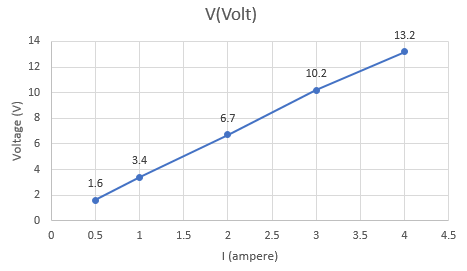Now the slope of this graph is the resistance
Therefore
$R= \frac {V_2 -V_1}{I_2 -I_1} = \frac {3.4 -1.6}{1 -.5}=3.6 \Omega$
$R= \frac {V_3 -V_2}{I_3 -I_2} = \frac {6.7 -3.4}{2- 1}=3.3 \Omega$
$R= \frac {V_4 -V_3}{I_4 - I_3} = \frac {10.2 -6.7}{3- 2}=3.5 \Omega$
$R= \frac {V_5 -V_4}{I_5 - I_4} = \frac {13.2 -10.2}{4- 3}=3 \Omega$
So Resistance can be calculated as average of these values
$R=\frac {3.6 + 3.3 + 3.5 + 3}{4}=3.35 \Omega$

Question 8.
When a 12 V battery is connected across an unknown resistor, there is a current of 2.5 mA in the circuit. Find the value of the resistance of the resistor.
Resistance (R) of a resistor is given by Ohm's law as,VIR
RV/I
Where,
Potential difference, V= 12 V
Current in the circuit, I= 2.5 mA = 2.5 x 10-3 A
$R= \frac {12}{2.5 \times 10^{-3}} = 4.8 \times 10^3= 4.8 K \Omega$
Therefore, the resistance of the resistor is 4.8 kΩ

Question 9.
A battery of 9 V is connected in series with resistors of 0.2 ?, 0.3 ?, 0.4 ? , 0.5 ? and 12 ?, respectively. How much current would flow through the 12 ? resistor?
We know that when the resistors are connected in series, same current through all the resistor and there is no current divisioning happening.
Current flow through the component is the same, given by Ohm’s law as
VIR
IV/R  -(1)
Where,
R is the equivalent resistance of resistances 0.2 Ω, 0.3 Ω, 0.4 Ω, 0.5 Ω and 12 Ω.
Now These are connected in series. Hence, the sum of the resistances will give the value of R.
R= 0.2 + 0.3 + 0.4 + 0.5 + 12 = 13.4 Ω
Potential difference, V= 9 V
So from equation –(1)
I= 9/13.4 = 0.671 A
Therefore, the current that would flow through the 12 Ω resistor is 0.671 A.

Question 10.
How many 176 $\Omega$ resistors (in parallel) are required to carry 5 A on a 220 V line?
For x number of resistors of resistance 176 Ω, the equivalent resistance of the resistors connected in parallel is given by Ohm's law as VIR
RV/I
Where,
Supply voltage, V= 220 V
Current, I = 5 A
Equivalent resistance of the combination = R, given as
1/Req = 1/R + +1/R +….x times
1/Req = x/176
Req = 176/x
Now from Ohm’s Law
RV/I
176/x=220/5
x=4
Therefore, four resistors of 176 Ω are required to draw the given amount of current.

Question 11.
Show how you would connect three resistors, each of resistance 6 ?, so that the combination has a resistance of (i) 9 ?, (ii) 4 ?.
This is quite interesting question; we need to look for the arrangement where desired result can be obtained.
Let’s check out few arrangement
a) If we connect the resistors in series, then the equivalent resistance will be the sum of the resistors, i.e., 6 Ω + 6 Ω + 6 Ω = 18 Ω
This is not what we desire
b) If we connect the resistors in parallel, then the equivalent resistance will be 6/2 = 3 Ω
This is not what we desire

c) Two resistor in parallel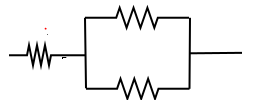Two 6 Ω resistors are connected in parallel. Their equivalent resistance will be
$\frac {1}{\frac {1}{6} + \frac {1}{6}} = \frac {6 \times 6}{6 +6}= 3 \Omega$
The third 6 Ω resistor is in series with 3 Ω. Hence, the equivalent resistance of the circuit is 6 Ω+ 3 Ω = 9 Ω.
This is what we desire in part (i)
(b) Two resistor in series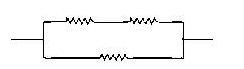Two 6 Ω resistors are in series. Their equivalent resistance will be the sum 6 + 6 = 12 Ω.
The third 6 Ω resistor is in parallel with 12 Ω. Hence, equivalent resistance will be
$\frac {1}{\frac {1}{12} + \frac {1}{6}} = \frac {12 \times 6}{12 + 6} = 4 \Omega$
Therefore, the total resistance is 4 Ω
This is what we desire in part (ii)

Question 12.
Several electric bulbs designed to be used on a 220 V electric supply line, are rated 10 W. How many lamps can be connected in parallel with each other across the two wires of 220 V line if the maximum allowable current is 5 A?
Supply voltage, V = 220 V
aximum allowable current, I = 5 A
Rating of an electric bulb P=10 watts
Total resistance of the circuit is
$R = \frac {V}{I} = \frac {220}{5} =44 \Omega$
Now resistance of each bulb
$R= \frac {V^2}{I} = \frac {220^2}{10= 4840 \Omega$
Let n be the number of bulbs in the circuit in parallel, then
$44= \frac {4840}{n}$
or
n=110

Question 13.
A hot plate of an electric oven connected to a 220 V line has two resistance coils A and B, each of 24 ? resistance, which may be used separately, in series, or in parallel. What are the currents in the three cases?
It is given in the question that,
Supply voltage, V= 220 V
Resistance of one coil, R= 24
(i) Coils are used separately
According to Ohm's law,
V= I1R1
Where,
I1 is the current flowing through the coil
$I_1= \frac {V}{R_1} = \frac {220}{24}= 9.166 A$
Therefore, 9.16 A current will flow through the coil when used separately.

(ii) Coils are connected in series
Total resistance for coils connected in series is given by expression
$R=R_1 + R_2 + R_3 + R_4 +.... R_n$
Total resistance of coils connected in series is
$R_2 = 24 + 24 = 48 \Omega$
According to Ohm's law,V = I2R2
Where, I2 is the current flowing through the series circuit
$I_2 = \frac {V}{R_2} = \frac {220}{48} = 4.58 A$
Therefore, 4.58 A current will flow through the circuit when the coils are connected in series.
(iii) Coils are connected in parallel and equivalent resistance of parallel combination is given as
$\frac {1}{R} = \frac {1}{R_1} + \frac {1}{R_2} + \frac {1}{R_3} + ....+ \frac {1}{R_n}$
Total resistance, R3 is given as =
$\frac {1}{\frac {1}{24} + \frac {1}{24}} =\frac {24}{2} = 12 \Omega$
According to Ohm's law,
V=I3R3
Where,
I3 is the current flowing through the circuit
$I_3 = \frac {V}{R_3} = \frac {220}{12} = 18.33 A$
Therefore, 18.33 A current will flow through the circuit when coils are connected in parallel.

Question 14.
Compare the power used in the 2 ? resistor in each of the following circuits: (i) a 6 V battery in series with 1 ? and 2 ? resistors, and (ii) a 4 V battery in parallel with 12 ? and 2 ? resistors.
(i) It is given in the question that,
Potential difference, V = 6 V
And 1Ω and 2Ω resistors are connected in series. Therefore, equivalent resistance of the circuit, R = 1Ω + 2Ω = 3Ω
According to Ohm's law,
V = IR
Where,
I is the current through the circuit
$I = \frac {6}{2} = 2A$
This current will flow through each component of the circuit because current is not divided between resistors in series combination of circuits. Hence, current flowing through the 2  resistor is 2 A. Power is given by the expression,
P= (I)2R = (2)2 x 2 = 8 W
(ii) Again from second part of the question it is given that,
Potential difference, V = 4 V
And 12Ω  and 2Ω  resistors are connected in parallel. The voltage across each component of a parallel circuit remains the same. Hence, the voltage across 2Ω resistor will be 4 V.
Power consumed by 2Ω  resistor is given by
$P= \frac {V^2}{R} = \frac {4^2}{2} = 8W$
Comparison between the power used in both the cases is
$\frac {P_1}{P_2} = \frac {8}{8} =1$

Question 15.
Two lamps, one rated 100 W at 220 V, and the other 60 W at 220 V, are connected in parallel to electric mains supply. What current is drawn from the line if the supply voltage is 220 V?
It is given in the question that both the bulbs are connected in parallel combination.
Therefore, potential difference across each of them will be 220 V,
This happens because no division of voltage takes place in a parallel circuit.
Current drawn by the bulb of rating 100 W is given by,
Power = Voltage x Current
$current = \frac {power}{voltage} = \frac {60}{220}$
Hence, current drawn from the line would be,
$I= \frac {100}{220} + \frac {60}{220} =.727 A$

Question 16.
Which uses more energy, a 250 W TV set in 1 hr, or a 1200 W toaster in 10 minutes?
Energy consumed by an electrical appliance is given by the expression
$H=Pt$
Where, P = power of the appliance and t = time.
Energy consumed by a TV set of power 250W in 1 hour = $250 W \times 3600 s = 9 \times 10^5$ J
Energy consumed by a toaster of power 1200 W in 10 minutes = 1200 ×600= 7.2×105 J
Therefore , the energy consumed by a 250 W TV set in 1 h is more than the energy consumed by a toaster of power 1200 W in 10 minutes.

Question 17.
An electric heater of resistance 8 ? draws 15 A from the service mains 2 hours. Calculate the rate at which heat is developed in the heater.
Rate of heat produced is given by the expression of power. Now power
$P=I^2R$
Where resistance of th electric heater is
$R=8 \Omega$
Current drawn is I=15A
Power P is,
$P=(15)^2 \times 8 = 1800 J/s$
Therefore heat is produced by the heater is at the rate of 1800J/s.

Question 18.
Explain the following.
(a) Why is the tungsten used almost exclusively for filament of electric lamps?
(b) Why are the conductors of electric heating devices, such as bread-toasters and electric irons, made of an alloy rather than a pure metal?
(c) Why is the series arrangement not used for domestic circuits?
(d) How does the resistance of a wire vary with its area of cross-section?
(e) Why are copper and aluminum wires usually employed for electricity transmission?
Here note that resistivity and melting point are two important factors here.
(a) The melting point and resistivity of tungsten are very high. It does not burn readily at a high temperature. The electric lamps glow at very high temperature. This is the reason behind using tungsten as heating element of electric bulbs.
(b) The conductors of electric heating devices such as bread toasters and electric irons are made of alloys because resistivity of alloys are more then that of metals. It produces large amount of heat.
(c) In series arrangement of resistances if the circuit breaks at one point then the flow of current would stop in whole circuit. This is the reason why we do not use series circuit in domestic circuits.
(d) Resistance of a wire is inversely proportional to its area of cross-section, that is when area of cross-section of wire increases , the resistance of the wire decreases and vice-versa.
(e) Copper and aluminum wires have low resistivity. They are good conductors of electricity. Hence they are u